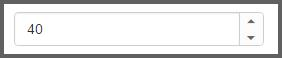# Getting Started

19 Oct 20162 minutes to read

This section explains briefly about how to create a NumericTextBox control in your application with PHP wrapper classes of EJ controls.

## Create your first NumericTextBox in PHP

You can create a PHP Project and add necessary scripts and styles with the help of the given PHP Getting Started Documentation.

You can render the PHP NumericTextBox control as show below.

• PHP
• ``````<?php
\$numeric = new EJ\NumericTextbox('numeric');
echo \$numeric->value(40)->width('250')->render();
?>``````

The following screenshot illustrates the output of the above code example.## Set the MinValue, MaxValue and value in NumericTextBox

You can set the “minValue”, ”maxValue” and “value” in Numeric text box for maintaining the range in Textboxes widgets. In this scenario, you have to enter the values between the given ranges. The following code example illustrates how to achieve this.

• PHP
• ``````<?php
\$numeric = new EJ\NumericTextbox('numeric');
echo \$numeric->value(40)->minValue(1)->maxValue(100)->width('250')->render();
?>``````

## Set the Strict Mode Option

You can set the “strict mode” option to restrict/allow entering values defined outside the range. The following code example illustrates how to set strict mode option. See API reference.

• PHP
• ``````<?php
\$numeric = new EJ\NumericTextbox("numeric");
echo \$numeric->value(40)->minValue(1)->maxValue(100)->width("250")->enableStrictMode(true)->render();
?>``````

Run the above code example and you can see that it allows entering a value exceeding the minValue and maxValue range mentioned in the Numeric textbox but it highlights the textbox with error class.